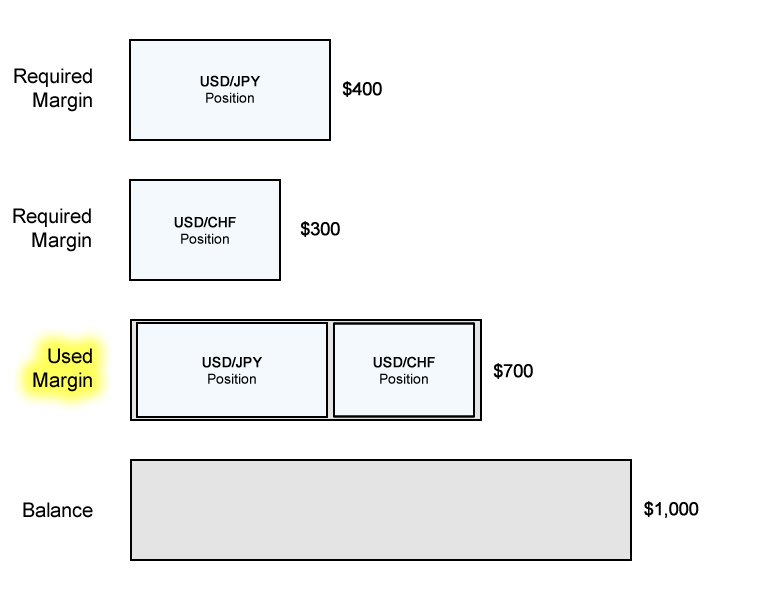What does “Used Margin” mean?

In order to understand what Used Margin is, we must first understand what Required Margin is.

Whenever you open a new position, a specific amount of Required Margin is set aside.

Required Margin was discussed in detail in the previous lesson, so if you don’t know what it is, please read our What is Margin? lesson first.

If you open more than one position at a time, each specific position will have its own Required Margin.

If you add up all of the Required Margin of all the positions that are open, the total amount is what’s called the Used Margin.

Used Margin is all the margin that’s “locked up” and can’t be used to open new positions.

This is margin is already being “used”. Hence the name, Used Margin.

While Required Margin is tied to a SPECIFIC trade, Used Margin refers to the amount of money you needed to deposit to keep ALL your trades open.

## Example: Open a long USD/JPY and USD/CHF position

Let’s say you’ve deposited \$1,000 in your account and want to open TWO positions:

1. Long USD/JPY and want to open 1 mini lot (10,000 units) position.
2. Long USD/CHF and want to open 1 mini lot (10,000 units) position.

The Margin Requirement for each currency pair is as follows:

 Currency Pair Margin Requirement USD/JPY 4% USD/CHF 3%

How much margin (“Required Margin”) will you need to open each position?

Since USD is the base currency for both currency pairs. a mini lot is 10,000 dollars, which means EACH position’s notional value is \$10,000.

Let’s now calculate the Required Margin for EACH position.

### USD/JPY Position

The Margin Requirement for USD/JPY is 4%. Assuming your trading account is denominated in USD, the Required Margin will be \$400.

```Required Margin = Notional Value x Margin Requirement

\$400 = \$10,000 x 0.04```

### USD/CHF Position

The Margin Requirement for USD/CHF is 3%.

Assuming your trading account is denominated in USD, the Required Margin will be \$300.

```Required Margin = Notional Value x Margin Requirement

\$300 = \$10,000 x 0.03```

```Used Margin = Sum of Required Margin from ALL open positions
Here’s a cool diagram of how Used Margin relates to Required Margin and Balance.